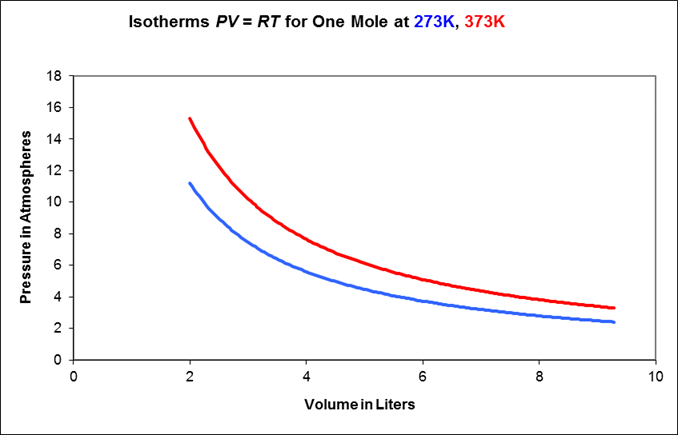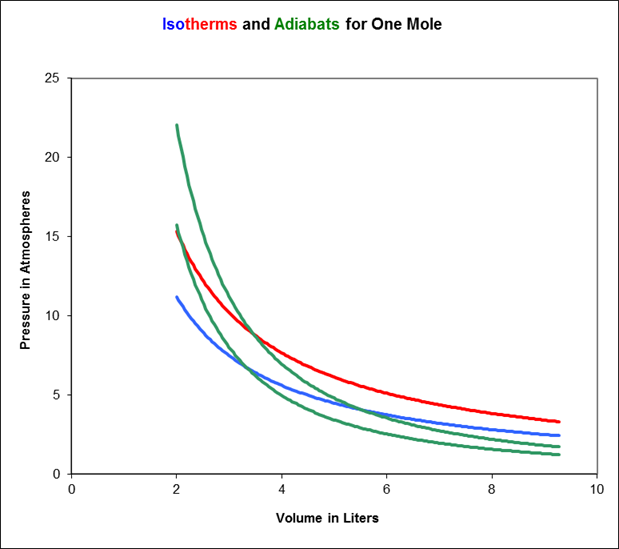# Ideal Gas Thermodynamics: Specific Heats, Isotherms, Adiabats

Michael Fowler

### Introduction: the Ideal Gas Model, Heat, Work and Thermodynamics

The Kinetic Theory picture of a gas (outlined in the previous lecture) is often called the Ideal Gas Model.  It ignores interactions between molecules, and the finite size of molecules. In fact, though, these only become important when the gas is very close to the temperature at which it become liquid, or under extremely high pressure.  In this lecture, we will be analyzing the behavior of gases in the pressure and temperature range corresponding to heat engines, and in this range the Ideal Gas Model is an excellent approximation.  Essentially, our program here is to learn how gases absorb heat and turn it into work, and vice versa.  This heat-work interplay is called thermodynamics.

Julius Robert Mayer was the first to appreciate that there is an equivalence between heat and mechanical work. The tortuous path that led him to this conclusion is described in an earlier lecture, but once he was there, he realized that in fact the numerical equivalence—how many Joules in one calorie in present day terminology—could be figured out easily from the results of some measurements of gas specific heat by French scientists.  The key was that they had measured specific heats both at constant volume and at constant pressure.  Mayer realized that in the latter case, heating the gas necessarily increased its volume, and the gas therefore did work in pushing to expand its container.  Having convinced himself that mechanical work and heat were equivalent, evidently the extra heat needed to raise the temperature of the gas at constant pressure was exactly the work the gas did on its container.  (Historical note: although he did the work in 1842, he didn’t publish until 1845, and at first miscalculated—but then gave a figure within 1% of the correct value of 4.2 joules per calorie.)

The simplest way to see what’s going on is to imagine the gas in a cylinder, held in by a piston, carrying a fixed weight, able to move up and down the cylinder smoothly with negligible friction.  The pressure on the gas is just the total weight pressing down divided by the area of the piston, and this total weight, of course, will not change as the piston moves slowly up or down: the gas is at constant pressure.### The Gas Specific Heats CV and CP

Consider now the two specific heats of this same sample of gas, let’s say one mole:

Specific heat at constant volume, $C V$ (piston glued in place),

Specific heat at constant pressure, $C P$ (piston free to rise, no friction).

In fact, we already worked out $C V$ in the Kinetic Theory lecture: at temperature $T,$ recall the average kinetic energy per molecule is $3 2 k B T,$ so one mole of gas$—$Avogadro’s number of molecules$—$will have total kinetic energy, which we’ll label internal energy,

$E int = 3 2 k B T⋅ N A = 3 2 RT.$

(In this simplest case, we are ignoring the possibility of the molecules having their own internal energy: they might be spinning or vibrating—we’ll include that shortly).

That the internal energy is $3 2 RT$ per mole immediately gives us the specific heat of a mole of gas in a fixed volume,

$C V = 3 2 R$

that being the heat which must be supplied to raise the temperature by one degree.

However, if the gas, instead of being in a fixed box, is held in a cylinder at constant pressure, experiment confirms that more heat must be supplied to raise the gas temperature by one degree.

As Mayer realized, the total heat energy that must be supplied to raise the temperature of the gas one degree at constant pressure is $3 2 k B$ per molecule plus the energy required to lift the weight.

The work the gas must do to raise the weight is the force the gas exerts on the piston multiplied by the distance the piston moves.

If the area of piston is $A,$ then the gas at pressure $P$ exerts force $PA.$

If on heating through one degree the piston rises a distance $Δ​ h$ the gas does work

$PA⋅Δ h=PΔV.$

Now, for one mole of gas, $PV=RT,$ so at constant $P$

$PΔV=RΔT.$

Therefore, the work done by the gas in raising the weight is just $RΔT,$ the specific heat at constant pressure, the total heat energy needed to raise the temperature of one mole by one degree,

$C P = C V +R.$

In fact, this relationship is true whether or not the molecules have rotational or vibrational internal energy.  (It’s known as Mayer’s relationship.)  For example, the specific heat of oxygen at constant volume

$C V ( O 2 )= 5 2 R$

and this is understood as a contribution of $3 2 R$ from kinetic energy, and $R$ from the two rotational modes of a dumbbell molecule (just why there is no contribution form rotation about the third axis can only be understood using quantum mechanics).  The specific heat of oxygen at constant pressure

$C P ( O 2 )= 7 2 R.$

It’s worth having a standard symbol for the ratio of the specific heats:

$C P C V =γ.$

### Tracking a Gas in the (P, V) Plane: Isotherms and Adiabats

An ideal gas in a box has three thermodynamic variables: $P,V,T.$  But if there is a fixed mass of gas, fixing two of these variables fixes the third from $PV=nRT$ (for $n$ moles). In a heat engine, heat can enter the gas, then leave at a different stage.  The gas can expand doing work, or contract as work is done on it.  To track what’s going on as a gas engine transfers heat to work, say, we must follow the varying state of the gas.  We do that by tracing a curve in the $( P, V )$ plane.

Supplying heat to a gas which consequently expands and does mechanical work is the key to the heat engine.  But just knowing that a gas is expanding and doing work is not enough information to follow its path in the $( P, V )$ plane.  The route it follows will depend on whether or not heat is being  supplied (or taken away) at the same time.  There are, however, two particular ways a gas can expand reversibly$—$meaning that a tiny change in the external conditions would be sufficient for the gas to retrace its path in the $( P, V )$ plane backwards.  It’s important to concentrate on reversible paths, because as Carnot proved and we shall discuss later, they correspond to the most efficient engines.  The two sets of reversible paths are the isotherms and the adiabats.

Isothermal behavior:   the gas is kept at constant temperature by allowing heat flow back and forth with a very large object (a “heat reservoir”) at temperature $T.$  From $PV=nRT,$ it is evident that for a fixed mass of gas, held at constant $T$  but subject to (slowly) varying pressure, the variables $P, V$ will trace a hyperbolic path in the $( P, V )$ plane.

This path, $PV=nR T 1 ,$ say is called the isotherm at temperature $T 1 .$   Here are two examples of isotherms:Adiabatic behavior:   “adiabatic” means “nothing gets through”, in this case no heat gets in or out of the gas through the walls.  So all the work done in compressing the gas has to go into the internal energy $E int .$As the gas is compressed, it follows a curve in the $( P, V )$ plane called an adiabat.  To see how an adiabat differs from an isotherm, imagine beginning at some point on the blue 273K isotherm on the above graph, and applying pressure so the gas moves to higher pressure and lower volume. Since the gas’s internal energy is increasing, but the number of molecules is staying the same, its temperature is necessarily rising, it will move towards the red curve, then above it.  This means the adiabats are always steeper than the isotherms

In the diagram below, we’ve added a couple of adiabats to the isotherms:What equation for an adiabat corresponds to $PV=nR T 1$ for an isotherm?

On raising the gas temperature by $ΔT,$ the change in the internal energy$—$the sum of molecular kinetic energy, rotational energy and vibrational energy (if any),

$Δ E int = C V ΔT.$

This is always true: whether or not the gas is changing volume is irrelevant, all that counts in $E int$ is the sum of the energies of the individual molecules (assuming as we do here that attractive or repulsive forces between molecules are negligible).

In adiabatic compression, all the work done by the external pressure goes into this internal energy, so

$−PΔV= C V ΔT.$

(Compressing the gas of course gives negative $ΔV,$ positive $Δ E int .$ )

To find the equation of an adiabat, we take the infinitesimal limit

$−PdV= C V dT,$

divide the left-hand side by $PV,$ the right-hand side by $nRT$ (since $PV=nRT,$

that’s OK) to find

$− R C V dV V = dT nT .$

Recall now that $C P = C V +nR,$ and $C P / C V =γ.$ It follows that

$nR C V = C P − C V C V =γ−1.$

Hence

$−( γ−1 ) ∫ dV V = ∫ dT T$

and integrating

$lnT+( γ−1 )lnV=const.$

from which the equation of an adiabat is

From $PV=nRT,$, the $P, V$ equation for an adiabat can be found by multiplying the left-hand side of this equation by the constant $PV/T,$  giving

where $γ= 5 3$ for a monatomic gas, $7 5$ for a diatomic gas.